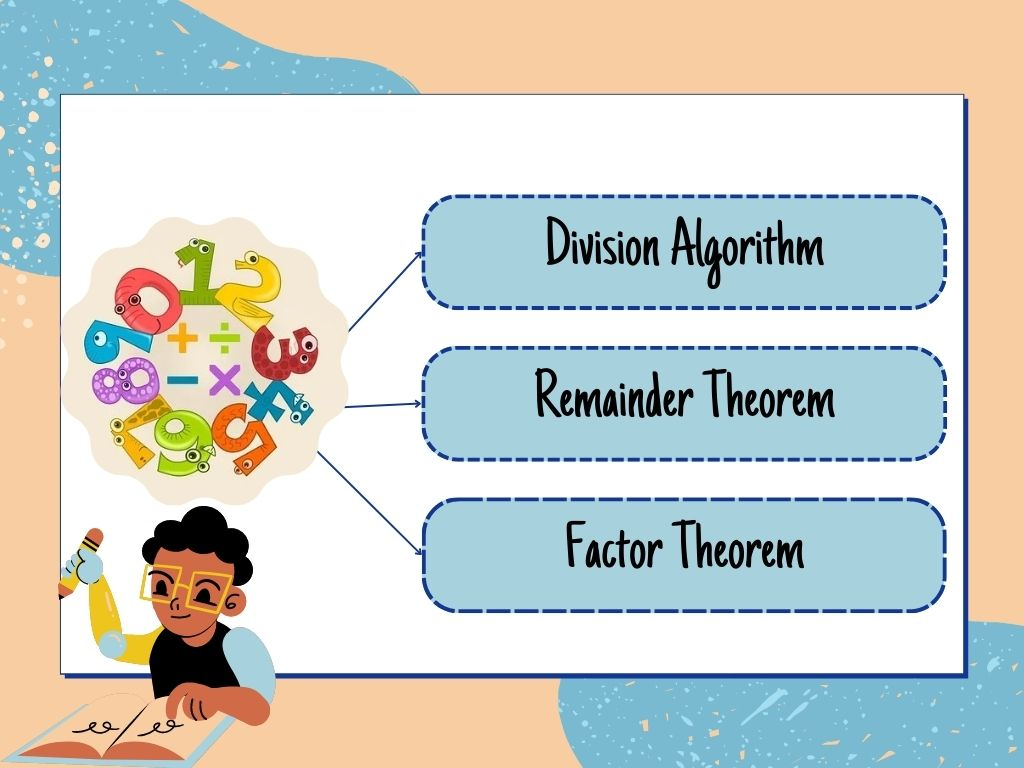## Division Algorithm, Remainder Theorem, and Factor Theorem Class 10th

Introduction Division Algorithm, Remainder Theorem, and Factor Theorem all three are related to Algebra. These three represent the relationship between the two functions. Division Algorithm If p(x) and g(x) are two such polynomials that the degree of p(x) is greater than g(x) and g(x) ≠ 0, and we divide p(x) by g(x) then we get two …